# Create a Gallery of Transformed Images

This example shows many properties of geometric transformations by applying different transformations to a checkerboard image.

A two-dimensional geometric transformation is a mapping that associates each point in a Euclidean plane with another point in a Euclidean plane. In these examples, the geometric transformation is defined by a rule that tells how to map the point with Cartesian coordinates (x, y) to another point with Cartesian coordinates (u, v). A checkerboard pattern is helpful in visualizing a coordinate grid in the plane of the input image and the type of distortion introduced by each transformation.

Create a sample checkerboard image using the `checkerboard` function. The image has rectangular tiles and four unique corners, which makes it easy to see how the checkerboard image gets distorted by geometric transformations. After you run this example once, try changing the image `I` to your favorite image.

```sqsize = 60; I = checkerboard(sqsize,4,4); imshow(I) title("Original")```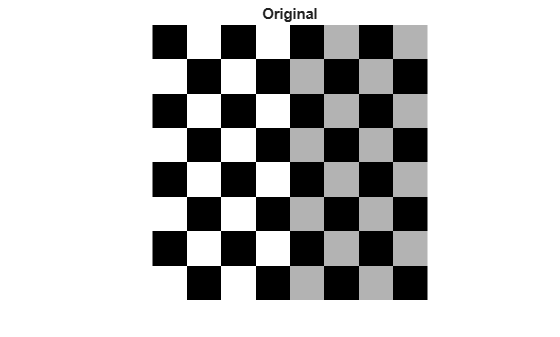Get the size of the image, and specify a fill value for the background.

```nrows = size(I,1); ncols = size(I,2); fill = 0.3;```

### Similarity Transformation

Similarity transformations can include rotation, isotropic scaling, and translation, but not reflection. Shapes and angles are preserved. Parallel lines remain parallel and straight lines remain straight.

Specify the rotation angle, scale factor, and translation amounts in the x and y directions. Then create a similarity geometric transformation object.

```scale = 1.2; angle = 40; tx = 0; ty = 0; t_sim = simtform2d(scale,angle,[tx ty]);```

Apply the similarity geometric transformation to the image and display the result.

```I_similarity = imwarp(I,t_sim,FillValues=fill); imshow(I_similarity); title("Similarity")```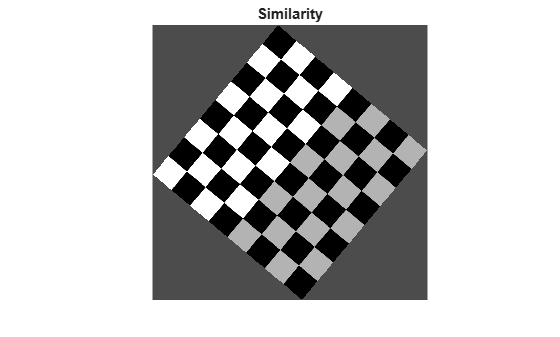If you change either `tx` or `ty` to a nonzero value, you will notice that it has no effect on the output image. If you want to see the coordinates that correspond to your transformation, including the translation, include spatial referencing information:

```[I_similarity,RI] = imwarp(I,t_sim,FillValues=fill); imshow(I_similarity,RI) axis on title("Similarity (Spatially Referenced)")```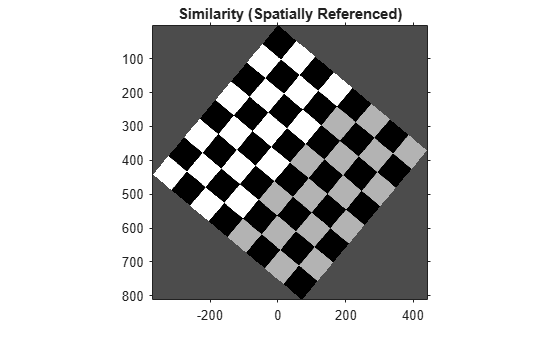Notice that passing the output spatial referencing object `RI` from `imwarp` reveals the translation. To specify what part of the output image you want to see, use the `OutputView` name-value argument in the `imwarp` function.

### Reflective Similarity Transformation

In a reflective similarity transformation, similar triangles map to similar triangles.

Specify the rotation angle, scale factor, and translation amounts in the x and y directions, and reflection coefficient. Specify the reflection coefficient `r` as -1 to perform reflection, and 1 otherwise. Create a 3-by-3 matrix that represents the transformation.

```scale = 1.5; angle = 10; tx = 0; ty = 0; r = -1; sc = scale*cosd(angle); ss = scale*sind(angle); A = [ sc r*ss tx; -ss r*sc ty; 0 0 1];```

Because reflective similarities are a subset of affine transformations, create an affine geometric transformation object from the geometric transformation matrix.

`t_reflective = affinetform2d(A);`

Apply the reflective similarity transformation to the image and display the result with the output spatial referencing information.

```[I_reflective,RI] = imwarp(I,t_reflective,FillValues=fill); imshow(I_reflective,RI) axis on title("Reflective Similarity")```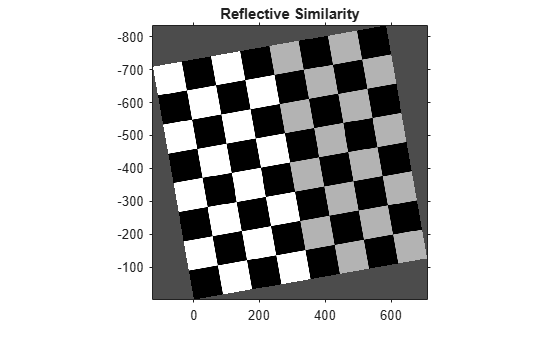### Affine Transformation

In an affine transformation, the x and y dimensions can be scaled or sheared independently and there can be translation, reflection, and rotation. Parallel lines remain parallel. Straight lines remain straight.

Specify a general affine transformation matrix. All six elements of the first and second columns can be different, and the third row must be [0 0 1]. Create an affine geometric transformation object from the geometric transformation matrix.

```A = [ 1 1 0; 0.3 2 0; 0 0 1]; t_aff = affinetform2d(A);```

Apply the generic affine transformation to the image and display the result with the output spatial referencing information.

```I_affine = imwarp(I,t_aff,FillValues=fill); imshow(I_affine) title("Affine")```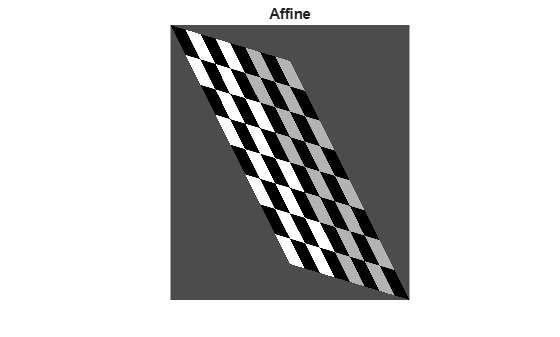### Projective Transformation

In a projective transformation, quadrilaterals map to quadrilaterals. Straight lines remain straight but parallel lines do not necessarily remain parallel.

Specify a general projective transformation matrix. All nine elements of the matrix can be different, with no constraints on the last row. Create a projective geometric transformation object from the geometric transformation matrix.

```A = [ 1 1 0; 0 1 0; 0.002 0.0002 1]; t_proj = projtform2d(A); ```

Apply the projective transformation to the image and display the result with the output spatial referencing information.

```I_projective = imwarp(I,t_proj,FillValues=fill); imshow(I_projective) title("Projective")```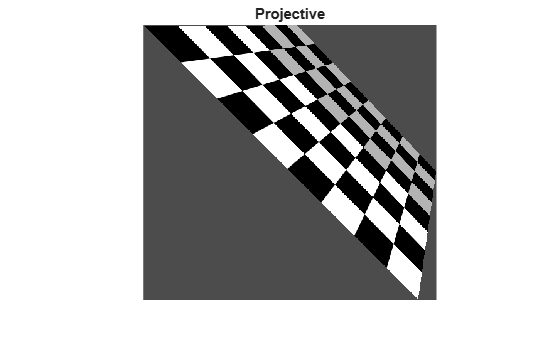### Piecewise Linear Transformation

In a piecewise linear transformation, affine transformations are applied separately to regions of the image. In this example, the top-left, top-right, and bottom-left points of the checkerboard remain unchanged, but the triangular region at the lower-right of the image is stretched so that the bottom-right corner of the transformed image is 50% further to the right and 20% lower than the original coordinate.

```movingPoints = [0 0; 0 nrows; ncols 0; ncols nrows;]; fixedPoints = [0 0; 0 nrows; ncols 0; ncols*1.5 nrows*1.2]; t_piecewise_linear = fitgeotform2d(movingPoints,fixedPoints,"pwl"); I_piecewise_linear = imwarp(I,t_piecewise_linear,FillValues=fill); imshow(I_piecewise_linear) title("Piecewise Linear")```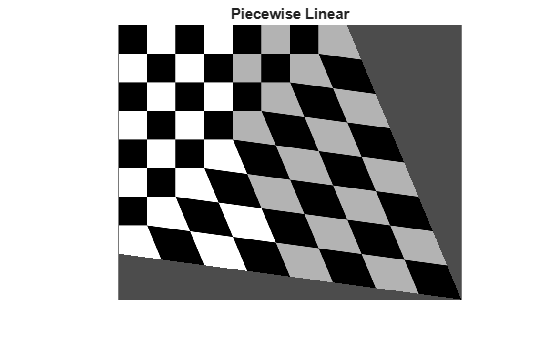### Sinusoidal Transformation

This example and the following two examples show how you can create an explicit mapping to associate each point in a regular grid (xi,yi) with a different point (ui,vi). This mapping is stored in a `geometricTransform2d` object, which used by `imwarp` to transform the image.

In this sinusoidal transformation, the x-coordinate of each pixel is unchanged. The y-coordinate of each row of pixels is shifted up or down following a sinusoidal pattern.

```a = ncols/12; % Try varying the amplitude of the sinusoid ifcn = @(xy) [xy(:,1), xy(:,2) + a*sin(2*pi*xy(:,1)/nrows)]; tform = geometricTransform2d(ifcn); I_sinusoid = imwarp(I,tform,FillValues=fill); imshow(I_sinusoid); title("Sinusoid")```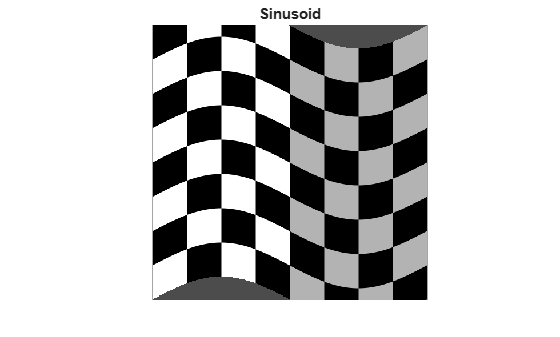### Barrel Transformation

Barrel distortion perturbs an image radially outward from its center. Distortion is greater farther from the center, resulting in convex sides.

First, define a function that maps pixel indices to distance from the center. Use the `meshgrid` function to create arrays of the x-coordinate and y-coordinate of each pixel, with the origin in the upper-left corner of the image.

`[xi,yi] = meshgrid(1:ncols,1:nrows);`

Shift the origin to the center of the image. Then, convert the Cartesian x- and y-coordinates to cylindrical angle (`theta`) and radius (`r`) coordinates using the `cart2pol` function. `r` changes linearly as distance from the center pixel increases.

```xt = xi - ncols/2; yt = yi - nrows/2; [theta,r] = cart2pol(xt,yt);```

Define the amplitude, `a`, of the cubic term. This parameter is adjustable. Then, add a cubic term to `r` so that `r` changes nonlinearly with distance from the center pixel.

```a = 1; % Try varying the amplitude of the cubic term. rmax = max(r(:)); s1 = r + r.^3*(a/rmax.^2);```

Convert back to the Cartesian coordinate system. Shift the origin back to the upper-right corner of the image.

```[ut,vt] = pol2cart(theta,s1); ui = ut + ncols/2; vi = vt + nrows/2;```

Store the mapping between (`xi`,`yi`) and (`ui`,`vi`) in a `geometricTransform2d` object. Use `imwarp` to transform the image according to the pixel mapping.

```ifcn = @(c) [ui(:) vi(:)]; tform = geometricTransform2d(ifcn); I_barrel = imwarp(I,tform,FillValues=fill); imshow(I_barrel) title("Barrel")```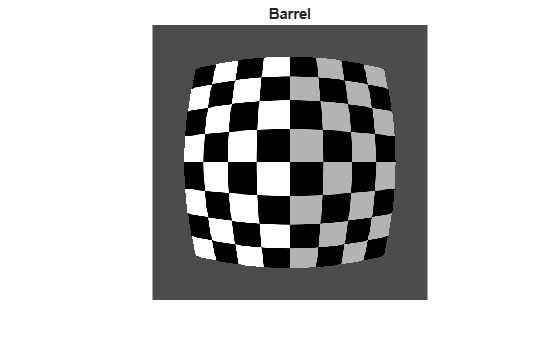### Pin Cushion Transformation

Pin-cushion distortion is the inverse of barrel distortion because the cubic term has a negative amplitude. Distortion is still greater farther from the center but the distortion appears as concave sides.

You can begin with the same `theta` and `r` values as for the barrel transformation. Define a different amplitude, b, of the cubic term. This parameter is adjustable. Then, subtract a cubic term to `r` so that `r` changes nonlinearly with distance from the center pixel.

```b = 0.4; % Try varying the amplitude of the cubic term. s = r - r.^3*(b/rmax.^2);```

Convert back to the Cartesian coordinate system. Shift the origin back to the upper-right corner of the image.

```[ut,vt] = pol2cart(theta,s); ui = ut + ncols/2; vi = vt + nrows/2;```

Store the mapping between (`xi`,`yi`) and (`ui`,`vi`) in a `geometricTransform2d` object. Use `imwarp` to transform the image according to the pixel mapping.

```ifcn = @(c) [ui(:) vi(:)]; tform = geometricTransform2d(ifcn); I_pin = imwarp(I,tform,FillValues=fill); imshow(I_pin) title("Pin Cushion")```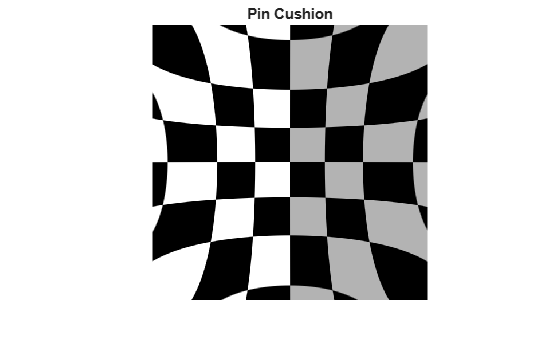### Summary: Display All Geometric Transformations of Checkerboard

```figure subplot(3,3,1),imshow(I),title("Original") subplot(3,3,2),imshow(I_similarity),title("Similarity") subplot(3,3,3),imshow(I_reflective),title("Reflective Similarity") subplot(3,3,4),imshow(I_affine),title("Affine") subplot(3,3,5),imshow(I_projective),title("Projective") subplot(3,3,6),imshow(I_piecewise_linear),title("Piecewise Linear") subplot(3,3,7),imshow(I_sinusoid),title("Sinusoid") subplot(3,3,8),imshow(I_barrel),title("Barrel") subplot(3,3,9),imshow(I_pin),title("Pin Cushion")```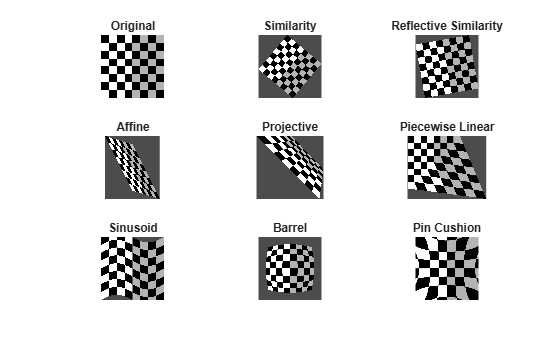Note that `subplot` changes the scale of the images being displayed.